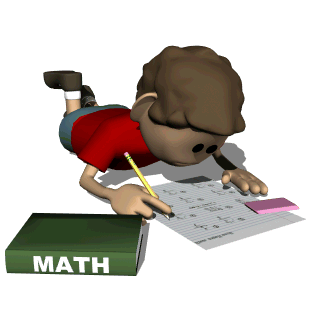• MathStudents will explore many mathematical concepts in the Third Grade.  We will use TERC - Investigations.  This is a standards based program of investigations that involve students in the exploration of major mathematical ideas.  Topics include: Addition, Subtraction, Data Analysis, 2-D and 3-D Geometry, Multiplication and Division, Fractions and Decimals, and Measurement.